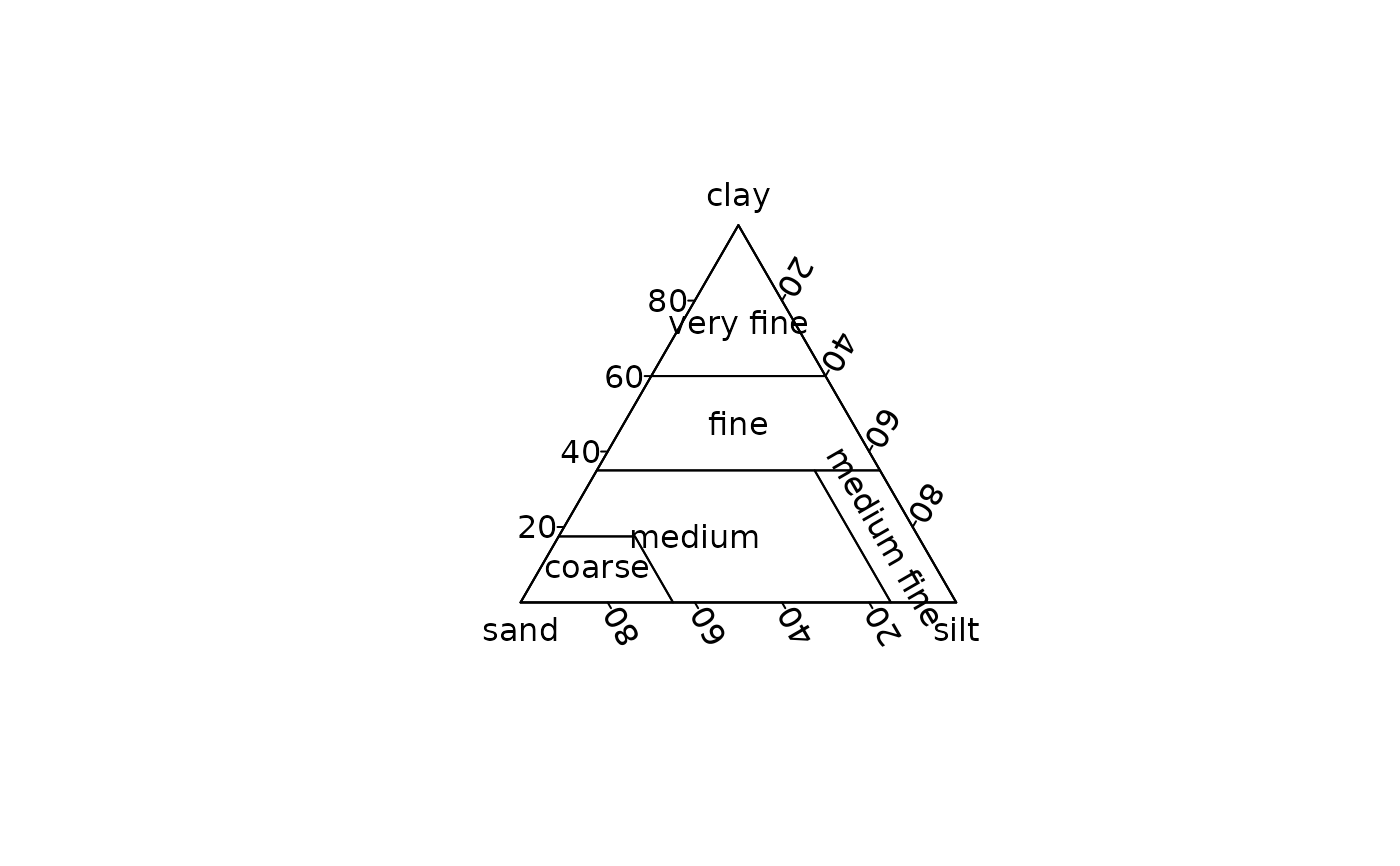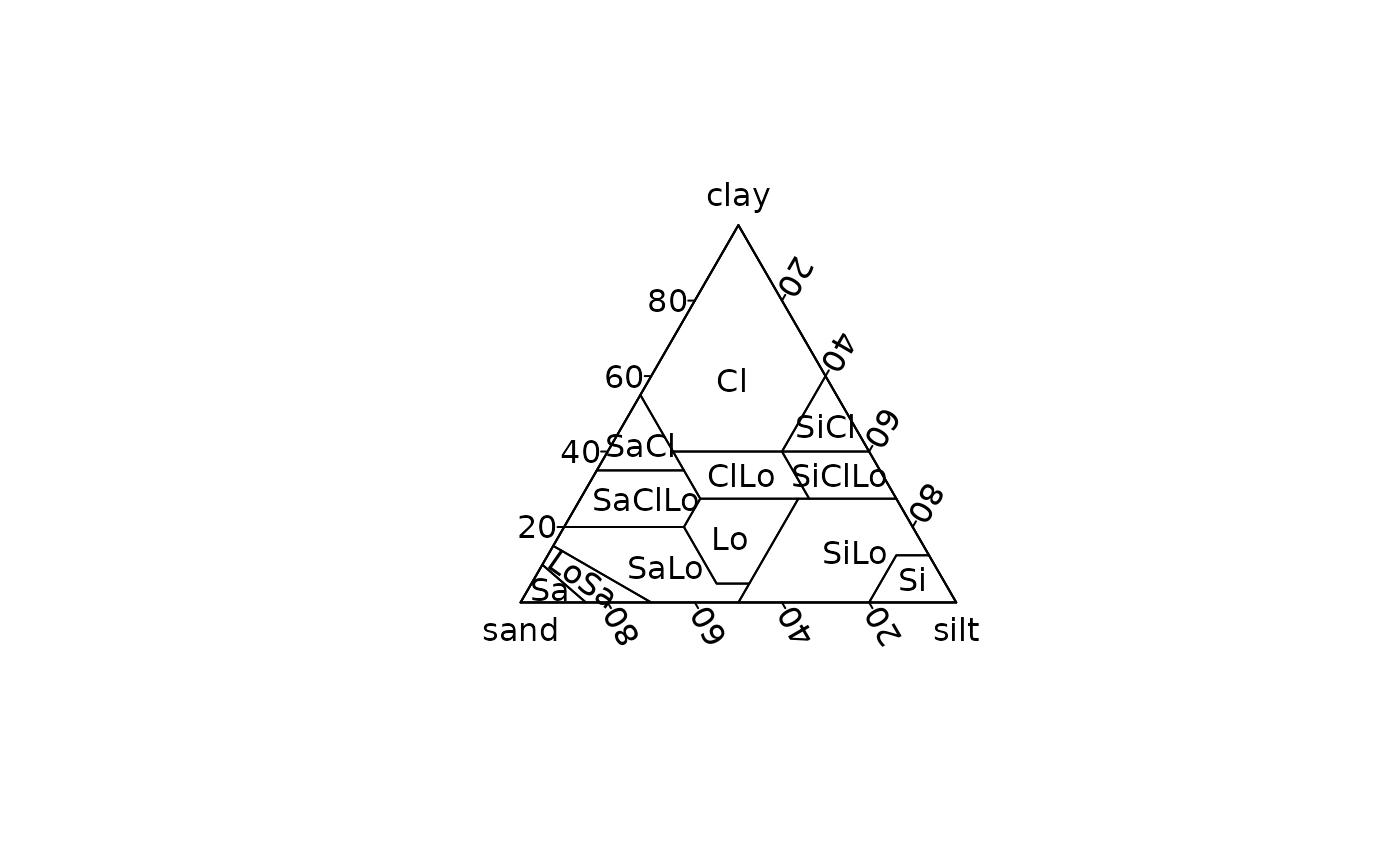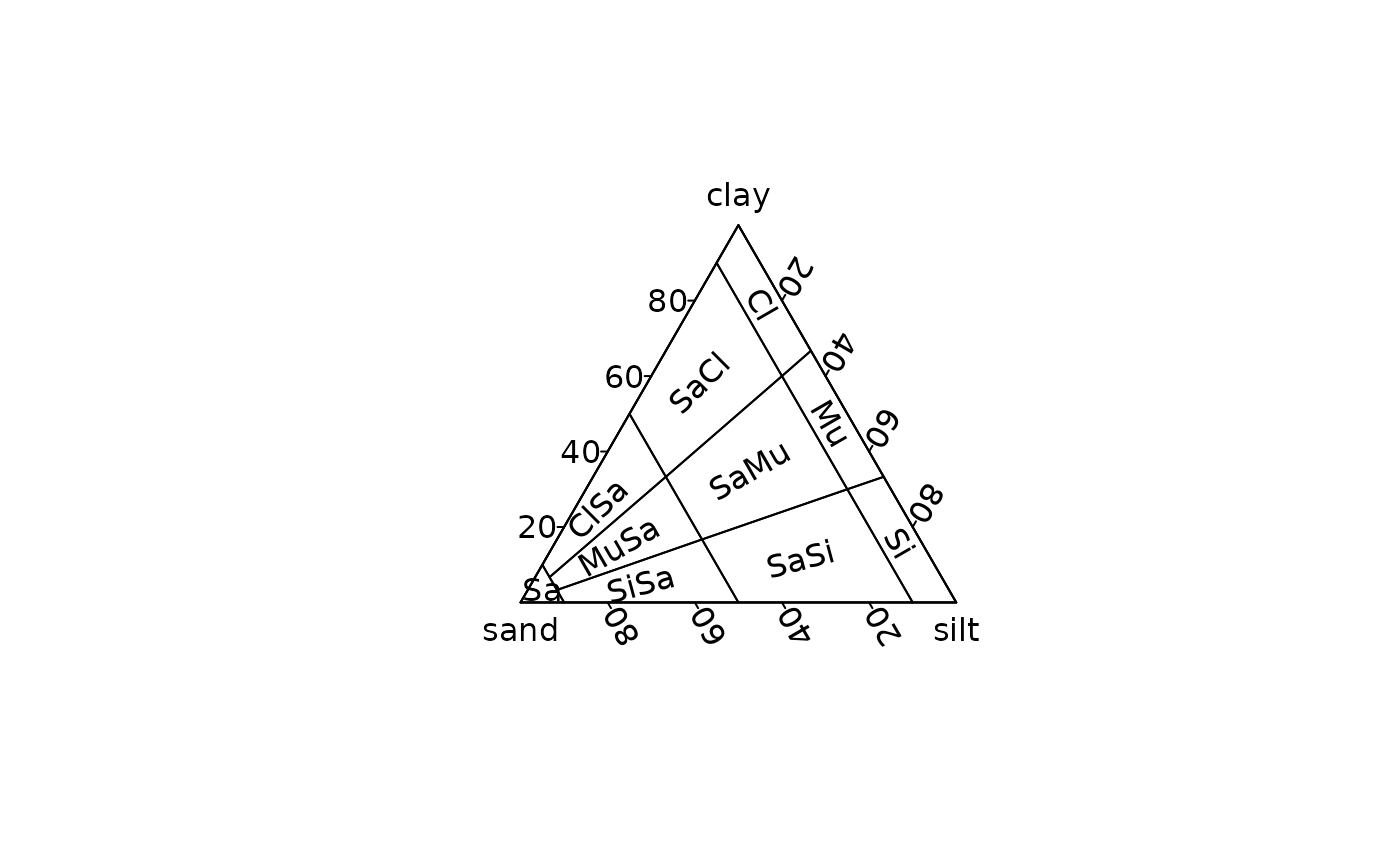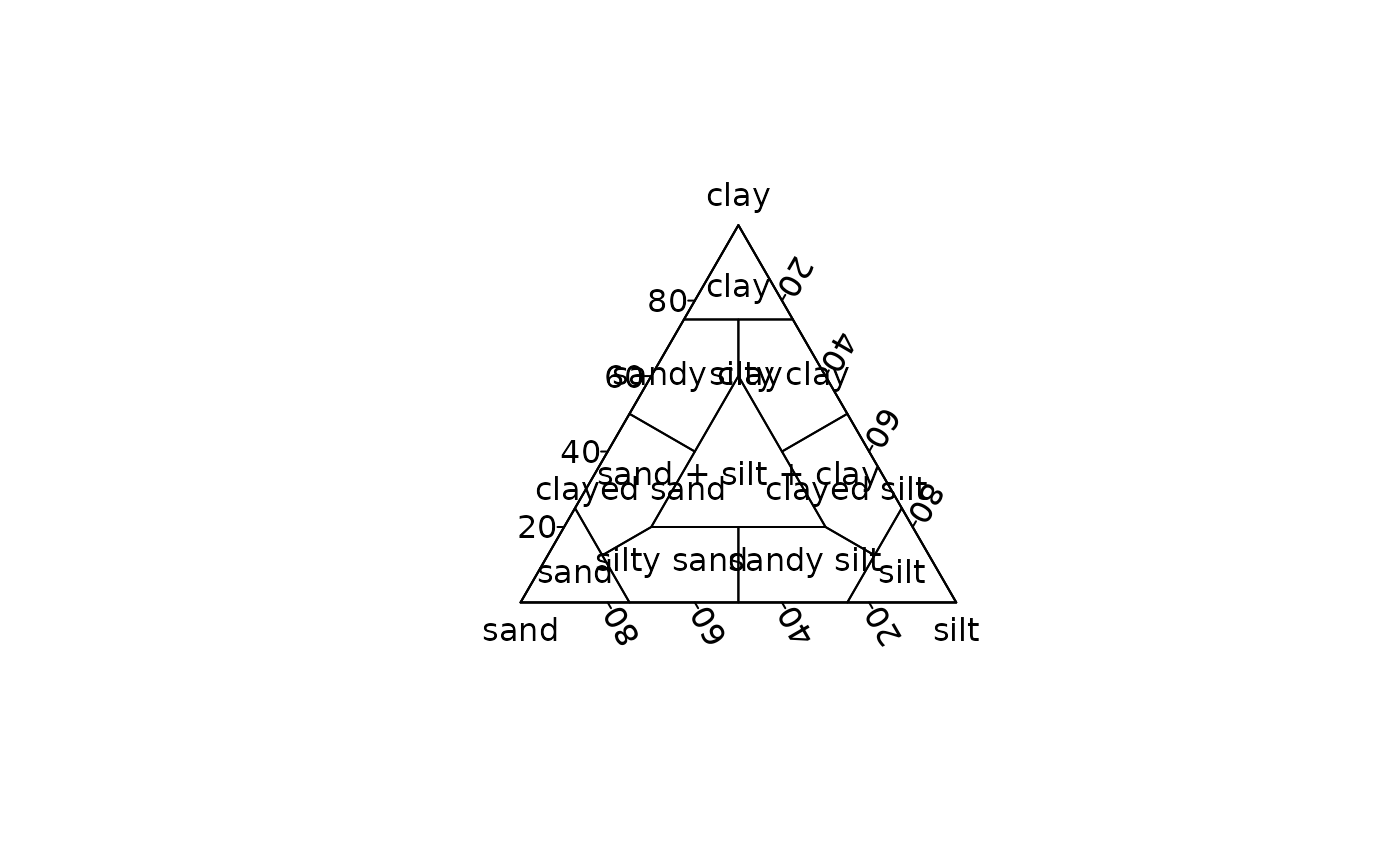Soil Texture Triangle

## Usage

triangle_soil_hypres(labels = TRUE, symbol = FALSE, ...)

triangle_soil_folk(labels = TRUE, symbol = FALSE, ...)

triangle_soil_shepard(labels = TRUE, symbol = FALSE, ...)

triangle_soil_usda(labels = TRUE, symbol = FALSE, ...)

## Arguments

labels

A logical scalar: should labels be displayed?

symbol

A logical scalar: should symbol be used instead of full labels? Only used if labels is TRUE.

...

Further arguments to be passed to graphics::polygon().

Other charts: triangle_phase_cas()

N. Frerebeau

## Examples

## HYPRES soil texture
ternary_plot(NULL, xlab = "sand", ylab = "silt", zlab = "clay")
triangle_soil_hypres()## USDA (1951) soil texture
ternary_plot(NULL, xlab = "sand", ylab = "silt", zlab = "clay")
triangle_soil_usda(symbol = TRUE)## Folk (1954) soil texture
ternary_plot(NULL, xlab = "sand", ylab = "silt", zlab = "clay")
triangle_soil_folk(symbol = TRUE)## Shepard (1954) soil texture
ternary_plot(NULL, xlab = "sand", ylab = "silt", zlab = "clay")
triangle_soil_shepard()